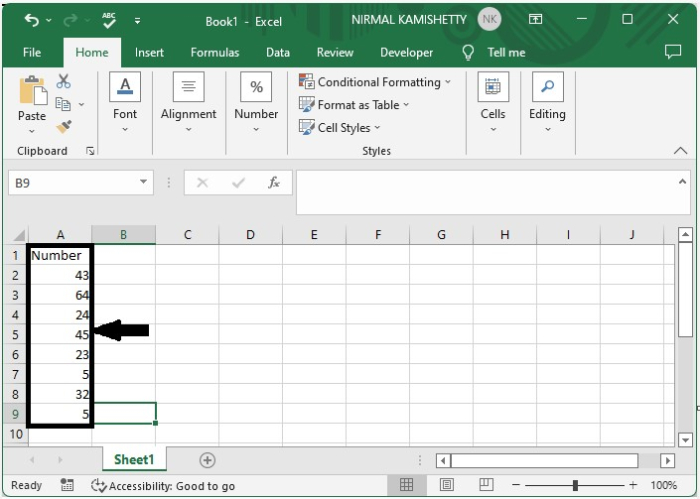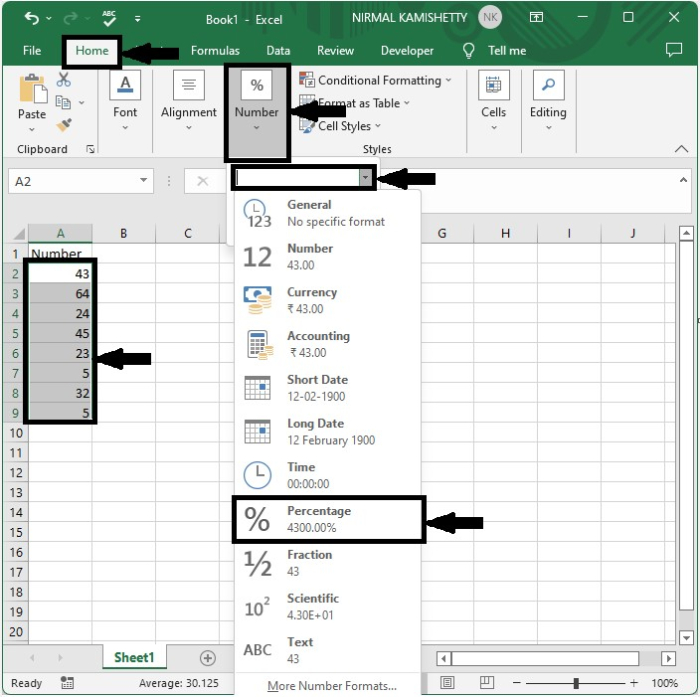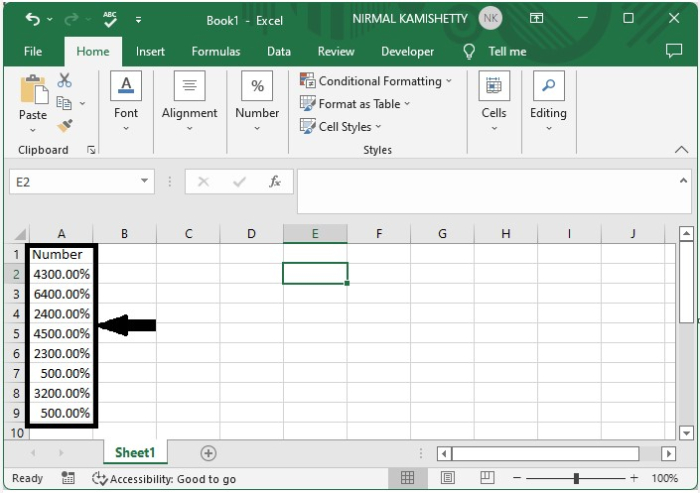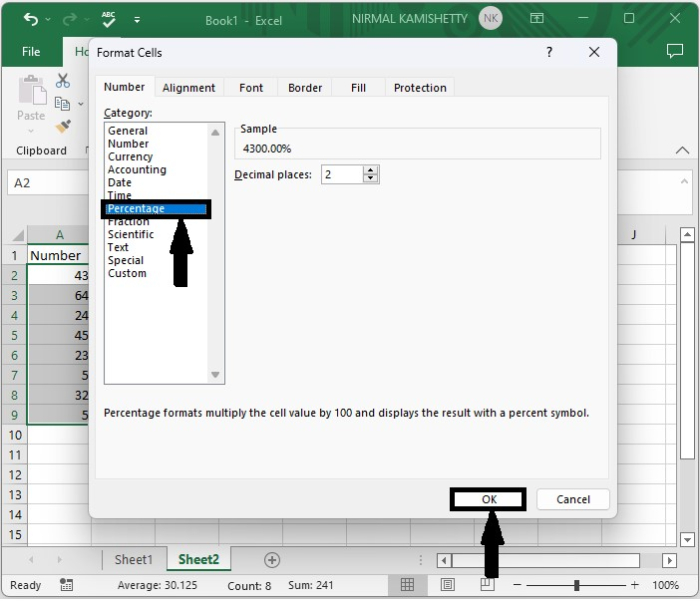# How to convert decimal to percentage in Excel?

There are many kinds of formats in Excel, like decimal number, percentage, date, and time. When we need to convert a decimal number to a percentage, we follow some simple steps. A decimal is a type of number with ten as its base value, and a percentage is the portion of the number that describes the ratio or fraction.

This tutorial will help you understand how we can convert a decimal to a percentage in Excel. We can complete this process using two methods. The first method is by changing the number format, and the other format is by formatting numbers. Let us see a brief process to solve this problem.

## Converting Decimal to Percentage using the Number Format

Here we will convert the values with the help of Font. Let's look at a simple procedure for converting a decimal to a percentage in Excel using the number format.

### Step 1

Let us consider an Excel sheet where the data in the sheet is similar to the data shown in the following screenshot.Now select the data. As shown below, click "home," then "number," and then "percentage" from the drop-down list.

Select data > home > Number > percentage### Step 2

The number will be displayed in percentage, and the value will be multiplied by 100, as shown in the following screenshot.## Converting Decimal to Percentage Using Format Cells

Here, we will first select the data and then use format to convert decimals to percentages. Let's go over a simple procedure for converting decimals to percentages in Excel using formatted cells.

### Step 1

Let us consider the same data that we used in the above example. Now select the data, then right-click on and select format cells to open the pop-up. Then, in the new pop-up window, click "percentage" and then "OK" to finish.

Select data > right click > format cells > percentage > ok## Conclusion

We used a simple example in this tutorial to show how you can convert decimal data to percentage form in Excel.

Updated on: 08-Feb-2023

451 Views